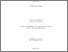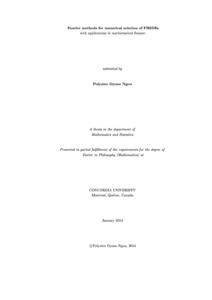Title:

# Fourier methods for numerical solution of FBSDEs with applications in mathematical finance

Oyono Ngou, Polynice (2014) Fourier methods for numerical solution of FBSDEs with applications in mathematical finance. PhD thesis, Concordia University.Preview
Text (application/pdf)
Oyono_NGou_PhD_S2014.pdf - Accepted Version
2MB

## Abstract

We present a Fourier analysis approach to numerical solution of forward-backward stochastic differential equations (FBSDEs) and propose two implementations. Using the Euler time discretization for backward stochastic differential equations (BSDEs), Fourier analysis allows to express the conditional expectations included in the time discretization in terms of Fourier integrals. The space discretization of these integrals then leads to expressions involving discrete Fourier transforms (DFTs) so that the FFT algorithm can be used. We quickly presents the convolution method on a uniform space grid. Locally, this first implementation produces a truncation error, a space discretization error and an additional extrapolation error. Even if the extrapolation error is convergent in time, the resulting absolute error may be high at the boundaries of the uniform space grid. In order to solve this problem, we propose a tree-like grid for the space discretization which suppresses the extrapolation error leading to a globally convergent numerical solution for the BSDE. The method is then extended to FBSDEs with bounded coefficients, reflected FBSDEs and higher order time discretizations of FBSDEs. Numerical examples from finance illustrate its performance.

Divisions: Concordia University > Faculty of Arts and Science > Mathematics and Statistics Thesis (PhD) Oyono Ngou, Polynice Concordia University Ph. D. Mathematics January 2014 Hyndman, Cody Forward-backward stochastic differential equations, Reflected FBSDEs, Numerical solution, Convolution, Fast Fourier transform. 978166 POLYNICE OYONO NGOU 16 Jun 2014 14:07 18 Jan 2018 17:46
All items in Spectrum are protected by copyright, with all rights reserved. The use of items is governed by Spectrum's terms of access.

Repository Staff Only: item control pageResearch related to the current document (at the CORE website)
Back to top